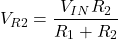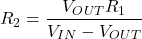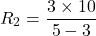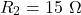# DC Voltage: What is it? (Circuit Symbol & Wire Color Codes)

Contents

## What is DC Voltage?

DC voltage stands for “Direct Current Voltage”. While this sounds confusing, the term “DC” has become used more broadly to refer to a system that has constant polarity. Hence DC voltage is a voltage that produces or would produce a DC current. Conversely, an AC voltage is a voltage that produces or would produce AC current.

DC in this context refers more broadly to quantities that don’t regularly change polarity or that have zero (or effectively zero) frequency. AC refers to quantities that regularly change polarity at a frequency above zero.

Voltage is the electric potential difference per unit charge between two points in an electric field. Electrical energy is generated from the movement and existence of charged particles known as electrons.

The movement of electrons creates a difference in potential energy between two points. We call this potential difference voltage.

There are two types of electrical energy; AC and DC. As mentioned, the voltage acquired from the DC source is known as DC voltage.

DC voltage has a constant value. And it is denoted as VDC. The frequency of DC voltage is zero (or near zero). Hence DC voltage systems will not change their polarity during operation.

## DC Voltage Symbol

The Unicode character-U+2393 “⎓” is used for the DC applications. Sometimes, it is also symbolled as a straight line.

In a circuit diagram, there are several DC sources available to get DC voltage. A battery is the most commonly used source for a DC voltage. The below figure shows the symbol of a battery.

Another circuit symbol commonly used for a DC voltage source is shown below.

The ideal DC voltage source has zero internal resistance. But an actual DC source will always have some amount of internal resistance.

In an ideal voltage source, the voltage drop across the source is zero. But in the case of a practical real-world voltage source, some amount of voltage drop occurs. This voltage drop increases as the current increases.

The VI characteristics of ideal and actual DC voltage source are as shown in the below figure.

## DC Voltage Wire Color Code

Wiring systems are color-coded to identify individual wires. This type of system is most helpful to identify wires during maintenance activities.

The term “DC power” is often used instead of “DC voltage” when speaking about wire color codes (note: DC power is not interchangeable with the term DC voltage. But “DC power” is used more broadly to denote that the circuit itself is DC, not AC).

### IEC DC Power Circuit Wire Color Codes

IEC (International Electrotechnical Commission) gives a standard for wiring code. The US National electrical code is different from IEC.

IEC Standards for DC voltage cable are as shown in the table below.

### US DC Power Circuit Wire Color Codes

US National Electrical code has discouraged the usage of ungrounded systems for safety reasons. Hence there are no wire color codes for the ungrounded DC system under the US National Electrical Code.

The recommended wire color codes for the grounded system under US National Electrical code are as given in the table below.

## Difference Between DC Voltage and AC Voltage

The major difference between DC voltage and AC voltage is as shown in the table below.

## How to Reduce DC Voltage

In certain scenarios we need to reduce DC voltage.

For example, let’s say a battery or cell is used to supply a DC voltage. You have a 9V battery, but the circuit requires only 3V. You’ll need to reduce the supply voltage to be able to use connect the battery to the circuit.

DC voltage can be reduced with the help of diodes and resistors.

### How to Reduce DC Voltage With Diodes

A diode conducts only when forward biased. Some voltage drop occurs across the terminals when a diode is connected.

The voltage drop in small silicon diodes is approximately 0.6-0.7V, germanium diode 0.25-0.3V, Schottky diode 0.2V.

We can further increase the voltage drop if we use the diodes in series with the load or circuit. This has been shown in the circuit below:

In our example, we need a 6V drop between the 3V load and the 9V battery. If we use silicon diodes, we need to add 4 or 5 diodes in the series, as shown in the above figure.

The anode of the first diode is connected with the positive terminal of the battery. And the anode of the second diode is connected with the cathode of the first diode. If you need an even create voltage drop, you can join a greater number of diodes in the same way.

### How to Reduce DC Voltage With Resistors

Resistors can be used to make a voltage divider circuit. Using this circuit, we can get any desired voltage drop.

You can get half of the input voltage by connecting two of the same resistance value in series.

But if you need a specific voltage drop, you can use the voltage drop formula below:From the above formula, you can get the voltage across the resistor R2. That is the voltage you need. But from this formula alone, you cannot find the resistance of R2.

For that, you have to assume the resistance of one resistor, input, and output voltage. Put these values in the below equation to find the resistance of R2.For example, you have a 5V battery as an input. And the circuit requires 3V output. Let’s assume you have one 10Ω resistor. Now, find the resistance of the second resistor R2.## How to Step-up DC Voltage

To step up the voltage is to increase the voltage level. You can step by DC voltage using a DC-DC power converter known as a boost Converter.

The boost converter is a class of Switched-Mode Power Supply (SMPS) that contains at least two semiconductor switches (diode/transistor) and one energy storage element (inductor/capacitor).

Filters are used to reduce voltage ripple. Usually, the filters are made of capacitors or a combination of capacitors and inductors. And it is connected at the load end or supply end of the converter.

In the operation of the boost converter, the power remains the same. As the voltage increase, the current decreases which make the power constant.

In AC power systems, a transformer is used to step up and step down the voltage level. This relies on an alternating current (AC) to create a changing flux and induce a current from the primary to the secondary side of the transformer via electromagnetic induction.

But because in DC power systems we have direct current (DC), which is constant, we cannot generate this changing flux. Hence a transformer will not work in DC power systems as there is no change in the magnetic field to induce voltage.

The boost converter used to step up the DC Voltage, and the buck converter used to step down DC voltage.

## How is DC Voltage Represented on Multimeters?

The multimeter is a measuring device that is used to measure the voltage, current, and resistance. The multimeters vary in size and features.

To measure the voltage, set the knob to the range of the given voltage. And connect probes to the voltage terminals in the multimeter.

We can measure the AC and DC voltage. To measure the DC voltage, set the multimeter at the DC sign.

Different voltage ranges given in some digital multimeters, like 2V, 20V, 200V, etc. If you need to measure the voltage below 20V, set the knob at 20V.

Want To Learn Faster? 🎓
Get electrical articles delivered to your inbox every week.
No credit card required—it’s 100% free.